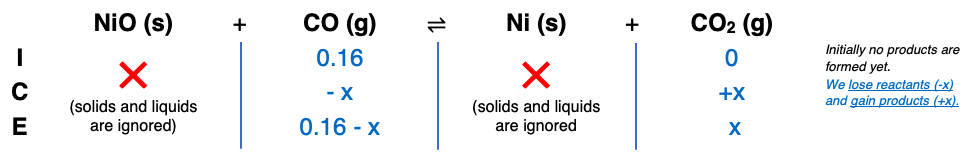# Problem: Consider the reaction: NiO(s) + CO(g) ⇌ Ni(s) + CO2(g); Kc =4000.0 at 1500 KWhen calculating the answer, do not round to the appropriate number of significant figures until the last calculation step. If a mixture of solid nickel(II) oxide and 0.16000 M carbon monoxide is allowed to come to equilibrium at 1500 K, what will be the equilibrium concentration of CO2?

###### FREE Expert Solution82% (341 ratings)###### Problem Details

Consider the reaction: NiO(s) + CO(g) ⇌ Ni(s) + CO2(g); Kc =4000.0 at 1500 K

When calculating the answer, do not round to the appropriate number of significant figures until the last calculation step. If a mixture of solid nickel(II) oxide and 0.16000 M carbon monoxide is allowed to come to equilibrium at 1500 K, what will be the equilibrium concentration of CO2?